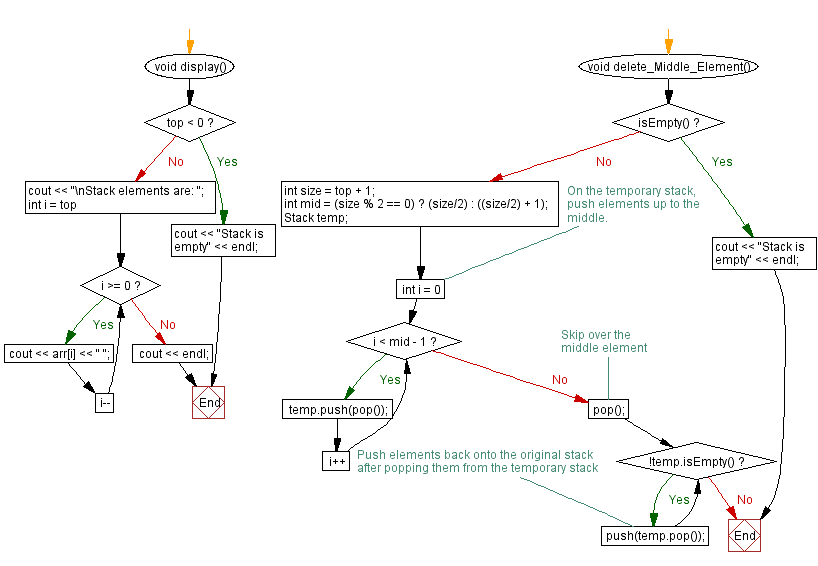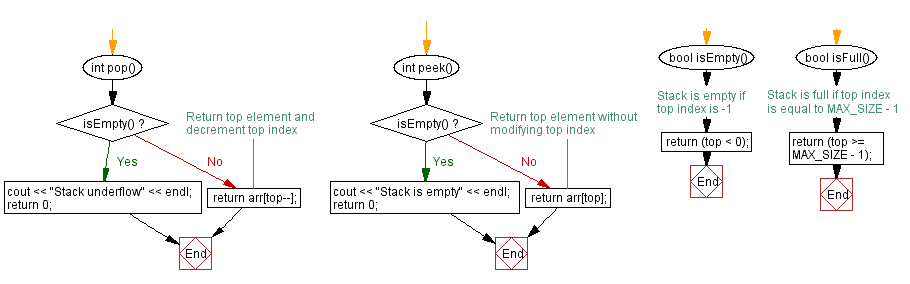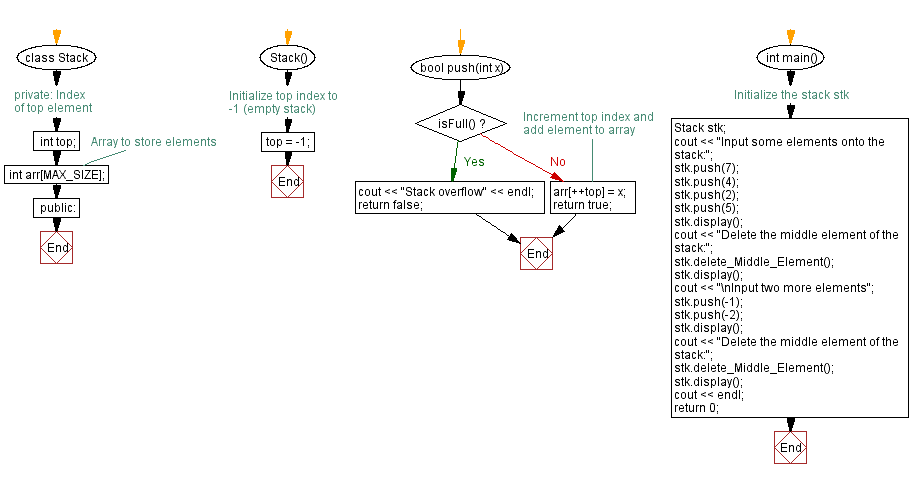﻿ C++ Delete the middle element of a stack (using an array)

# C++ Stack Exercises: Delete the middle element of a stack (using an array)

## C++ Stack: Exercise-9 with Solution

Write a C++ program to delete the middle element of a stack (using an array).

When the number of elements is odd, the middle element is at position (size/2) + 1. When the number of elements is even, there are two middle elements at positions size/2 and (size/2) + 1.

Test Data:
Input some elements onto the stack:
Stack elements are: 5 2 4 7
Delete the middle element of the stack:
Stack elements are: 5 4 7

Sample Solution:

C++ Code:

``````#include <iostream>

using namespace std;
#define MAX_SIZE 15 // Maximum size of stack

class Stack {
private:
int top; // Index of top element
int arr[MAX_SIZE]; // Array to store elements
public:
Stack() {
top = -1; // Initialize top index to -1 (empty stack)
}

bool push(int x) {
if (isFull()) {
cout << "Stack overflow" << endl;
return false;
}
// Increment top index and add element to array
arr[++top] = x;
return true;
}

int pop() {
if (isEmpty()) {
cout << "Stack underflow" << endl;
return 0;
}
return arr[top--];
}

int peek() {
if (isEmpty()) {
cout << "Stack is empty" << endl;
return 0;
}
return arr[top];
}

bool isEmpty() {
// Stack is empty if top index is -1
return (top < 0);
}

bool isFull() {
// Stack is full if top index is equal to MAX_SIZE - 1
return (top >= MAX_SIZE - 1);
}

void display() {
if (top < 0) {
cout << "Stack is empty" << endl;
return;
}
cout << "\nStack elements are: ";
for (int i = top; i >= 0; i--)
cout << arr[i] << " ";
cout << endl;
}

void delete_Middle_Element() {
if (isEmpty()) {
cout << "Stack is empty" << endl;
return;
}

int size = top + 1;
int mid = (size % 2 == 0) ? (size/2) : ((size/2) + 1);

Stack temp;
//On the temporary stack, push elements up to the middle.
for (int i = 0; i < mid - 1; i++) {
temp.push(pop());
}
// Skip over the middle element
pop();
while (!temp.isEmpty()) {
//Push elements back onto the original stack
//after popping them from the temporary stack
push(temp.pop());
}
}
};

int main() {
//Initialize the stack stk
Stack stk;
cout << "Input some elements onto the stack:";
stk.push(7);
stk.push(4);
stk.push(2);
stk.push(5);
stk.display();
cout << "Delete the middle element of the stack:";
stk.delete_Middle_Element();
stk.display();
cout << "\nInput two more elements";
stk.push(-1);
stk.push(-2);
stk.display();
cout << "Delete the middle element of the stack:";
stk.delete_Middle_Element();
stk.display();
cout << endl;
return 0;
}

``````

Sample Output:

```Input some elements onto the stack:
Stack elements are: 5 2 4 7
Delete the middle element of the stack:
Stack elements are: 5 4 7

Input two more elements
Stack elements are: -2 -1 5 4 7
Delete the middle element of the stack:
Stack elements are: -2 -1 4 7
```

Flowchart:CPP Code Editor: